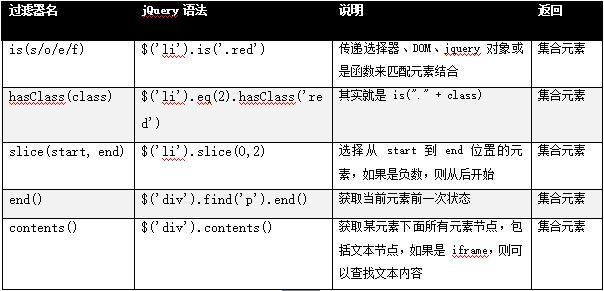020-38289118# {HTML5}过滤选择器-第五节-其他方法

​jQuery 在选择器和过滤器上，还提供了一些常用的方法，方便我们开发时灵活使用。\$('.red').is('li');                         //true，选择器，检测 class 为是否为 red

\$('.red').is(\$('li'));                      //true，jQuery 对象集合，同上

\$('.red').is(\$('li').eq(2));                 //true，jQuery 对象单个，同上

\$('.red').is(\$('li').get(2));                //true，DOM 对象，同上

\$('.red').is(function () {                 //true，方法，同上

return \$(this).attr('title') == '列表 3';  //可以自定义各种判断

}));

\$('li').eq(2).hasClass('red');            //和 is 一样，只不过只能传递 class

\$('li').slice(0,2).css('color', 'red');         //前三个变成红色

\$("div").find("p").end().get(0);                   //返回 div 的原生 DOM

\$('div').contents().size();                       //返回子节点(包括文本)数量

\$('li').filter('.red').css('background','#ccc');           //选择 li 的 class 为 red 的元素

\$('li').filter('.red, :first, :last').css('background','#ccc');    //增加了首尾选择

//特殊要求函数返回

\$('li').filter(function () {

return \$(this).attr('class') == 'red' && \$(this).attr('title') == '列表 3';

}).css('background', '#ccc');十三年老品牌
QQ咨询：450959328 微信咨询：togogozhong 咨询电话：020-38289118 咨询网站客服：在线客服